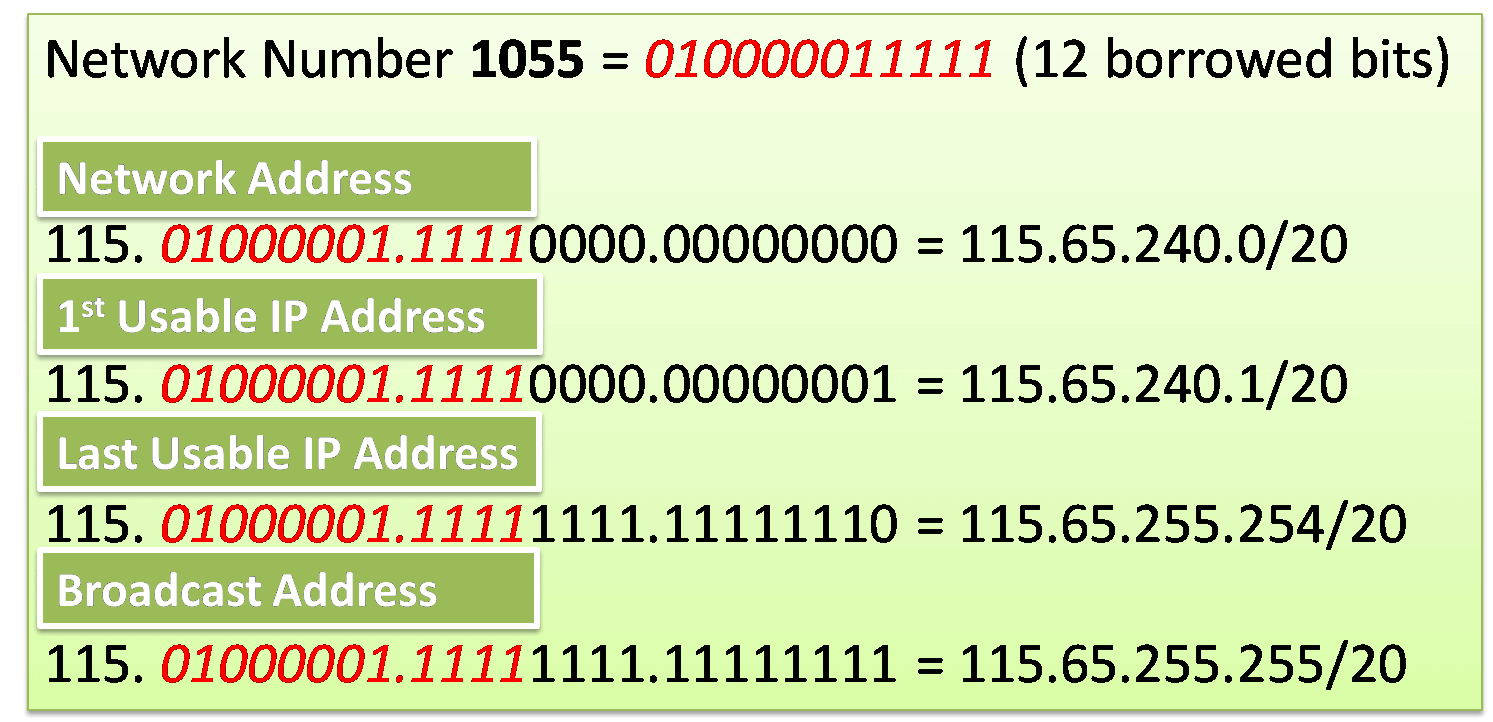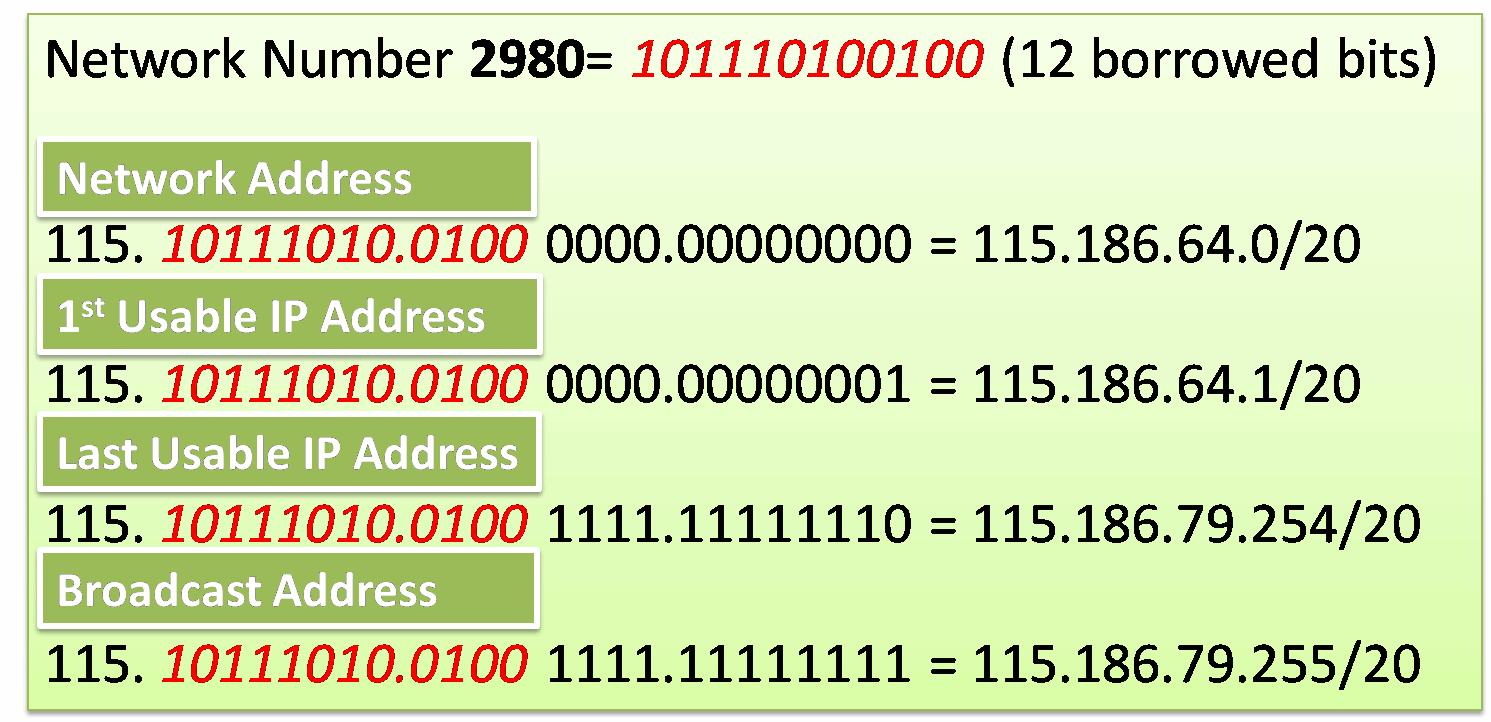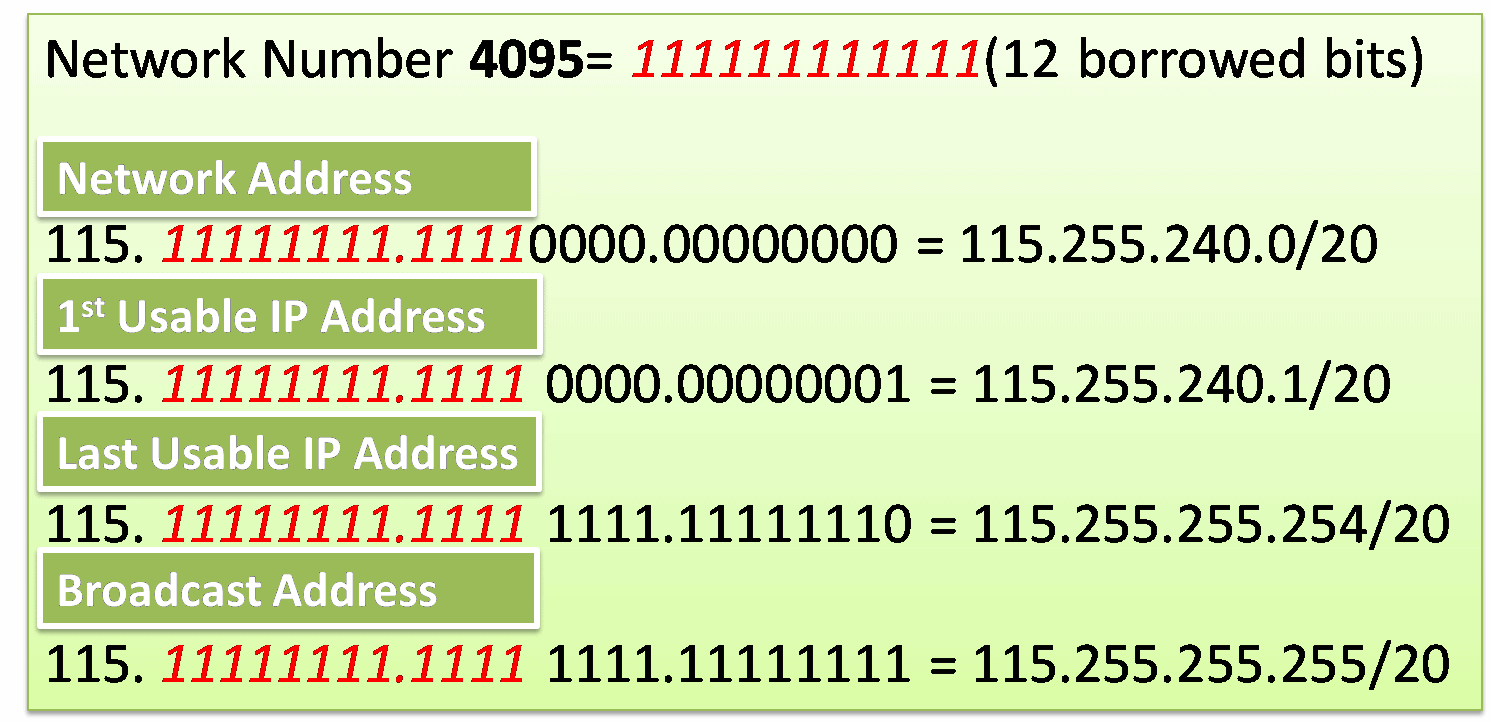## Creating 4000 Subnets from a /8 Prefix

Some organizations need more subnets. For example, a small ISP requires 4000 subnets for its clients. Each client required an abundance of space in the host portion to create their own subnets. The network address 115.0.0.0/8 has a default subnet mask of 255.0.0.0 or /8 prefix. The /8 prefix means that there are 24 host bits available to borrow.

To create subnets we must borrow bits from the host portion of the IP address from left to right with the first available host bit, we will borrow a single bit at a time until we reach the number of bits necessary to create 4000 subnets. We need to borrow 12 bits to create 4096 subnets. So, for borrowing 12 bits, we need 8 bits in the second octet and 4 extra bits from the third octet to borrow.

The resulting subnet mask for all sub-network convert from  (255.0.0.0  – 11111111.00000000.00000000.00000000)  to (255.255.240.0  – 11111111.11111111.11110000.00000000) or a /20 prefix.

The network address of /8 network is 115.0.0.0/8 and the broadcast address is 255.0.0.0 (11111111.00000000.00000000.00000000). The resulting subnets of borrowing 12 bits creating subnets from 115.00000000.000000000.000000000 /20 to 10.11111111.11110000.000000000 /20 (115.0.0.0/20 to 115.255.240.0/20. Each sub-network containing 2096 hosts. The Sub-network are starting from 0 to 4095 (4096 sub-networks). So how we can find the address range of any sub-network.? There are numerous ways to find the address range of any sub-network. Here we will find the address range for any sub-network using the sub-network number. There are five steps to find the address ranges for any sub-network which is following

1. Convert the network number into binary.
2. Place the binary digits into borrowed bits positions.
3. All bits in the host portion will be 0 in the host portion.
4. For the first usable IP address, all bits in the host portion will be 0, except the most right bit which should be 1.
5. For the last usable IP address, all bits will be 1’s except the rightmost bit, which should be 0.
6. All bits should be changed to 1 in the host portion of the broadcast address.

#### Examples of finding the network range for any subnetwork of the above mention /8 prefix network

Example-1 – fined the address range for network number 1055.For finding network number 1055’s address range, first of all, we required to find the binary of 1055 which is 10000011111.  It is 11 bits and we have borrowed 12 bits, so we can add 0 at the leftmost side which is 010000011111. After converting 1055 into binary place the 12 bits value into the places of borrowed bits in the 2nd and 3rd octet. Now follow the rules to find the network address, broadcast address, 1st and last usable IP addresses.

Example-2 – Let us fined the address range for network number 2040.Example-3 – fined the address range for network number 2980.Example-4 – fined the address range for network number 4025Example-5 – Let Us fined the address information for last subnet 4095.You can see that borrowed bits in the network portion are maximum now. Because 4095 is the last sub-network.